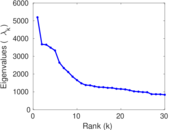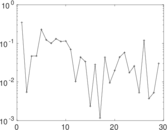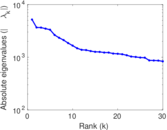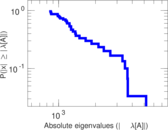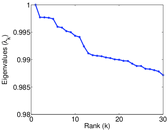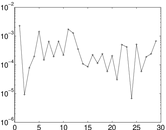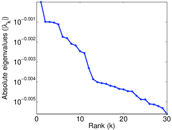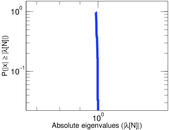# Wikibooks edits (en)

This is the bipartite edit network of the English Wikibooks. It contains users and pages from the English Wikibooks, connected by edit events. Each edge represents an edit. The dataset includes the timestamp of each edit.

 Code `ben` Internal name `edit-enwikibooks` Name Wikibooks edits (en) Data source http://dumps.wikimedia.org/ AvailabilityDataset is available for download Consistency checkDataset passed all tests Category Authorship network Dataset timestamp 2017-10-20 Node meaning User, article Edge meaning Edit Network formatBipartite, undirected Edge typeUnweighted, multiple edges Temporal dataEdges are annotated with timestamps

## Statistics

 Size n = 328,993 Left size n1 = 79,268 Right size n2 = 249,725 Volume m = 2,233,443 Unique edge count m̿ = 766,272 Wedge count s = 5,255,623,618 Claw count z = 83,438,718,750,347 Cross count x = 1,105,380,470,247,386,240 Square count q = 1,523,983,107 4-Tour count T4 = 33,216,173,564 Maximum degree dmax = 106,575 Maximum left degree d1max = 106,575 Maximum right degree d2max = 12,113 Average degree d = 13.577 4 Average left degree d1 = 28.175 8 Average right degree d2 = 8.943 61 Average edge multiplicity m̃ = 2.914 69 Size of LCC N = 313,096 Diameter δ = 13 50-Percentile effective diameter δ0.5 = 3.658 08 90-Percentile effective diameter δ0.9 = 4.949 17 Median distance δM = 4 Mean distance δm = 4.177 34 Gini coefficient G = 0.815 331 Balanced inequality ratio P = 0.164 954 Left balanced inequality ratio P1 = 0.116 142 Right balanced inequality ratio P2 = 0.208 526 Relative edge distribution entropy Her = 0.800 517 Power law exponent γ = 2.491 28 Tail power law exponent γt = 2.251 00 Tail power law exponent with p γ3 = 2.251 00 p-value p = 0.835 000 Left tail power law exponent with p γ3,1 = 2.011 00 Left p-value p1 = 0.911 000 Right tail power law exponent with p γ3,2 = 2.511 00 Right p-value p2 = 0.839 000 Degree assortativity ρ = −0.209 422 Degree assortativity p-value pρ = 0.000 00 Spectral norm α = 5,192.56 Algebraic connectivity a = 0.009 251 46 Spectral separation |λ1[A] / λ2[A]| = 1.413 58 Controllability C = 243,214 Relative controllability Cr = 0.746 204

## Plots

### Fruchterman–Reingold graph drawing### Degree distribution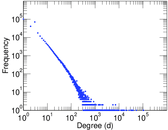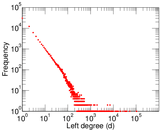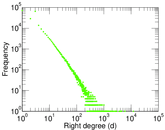### Cumulative degree distribution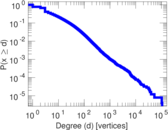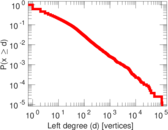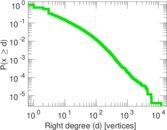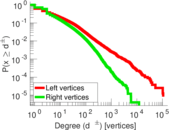### Lorenz curve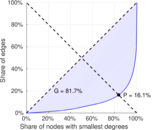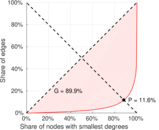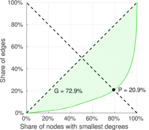### Spectral distribution of the adjacency matrix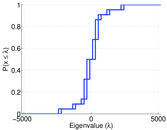### Spectral distribution of the normalized adjacency matrix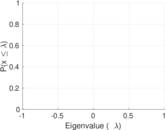### Spectral distribution of the Laplacian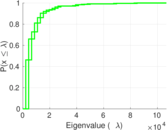### Spectral graph drawing based on the adjacency matrix### Spectral graph drawing based on the Laplacian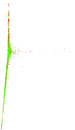### Spectral graph drawing based on the normalized adjacency matrix### Degree assortativity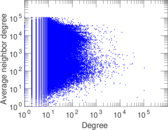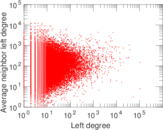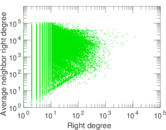### Zipf plot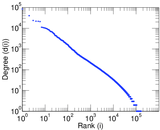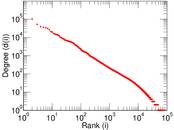### Hop distribution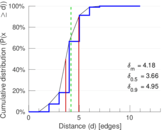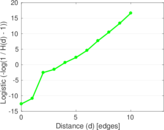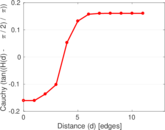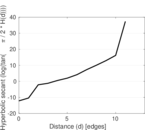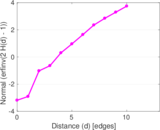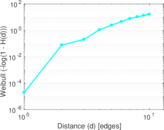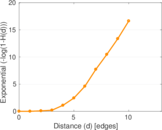### Delaunay graph drawing### Edge weight/multiplicity distribution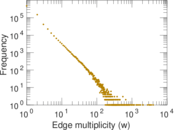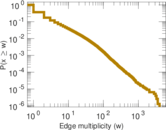### Temporal distribution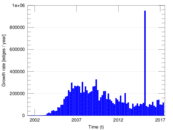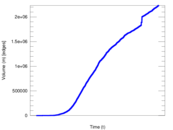### Diameter/density evolution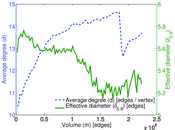### Inter-event distribution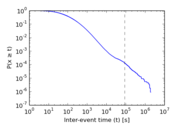### Node-level inter-event distribution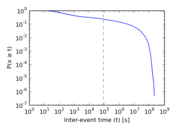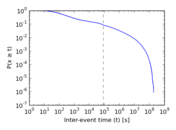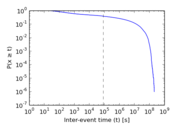### Matrix decompositions plots